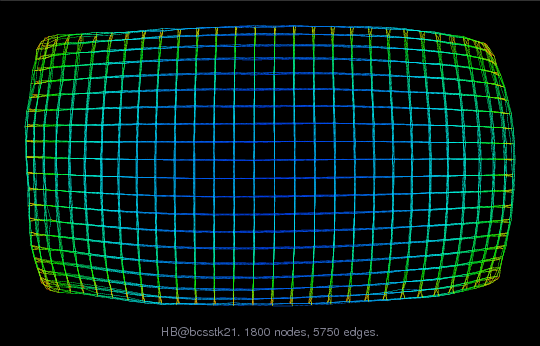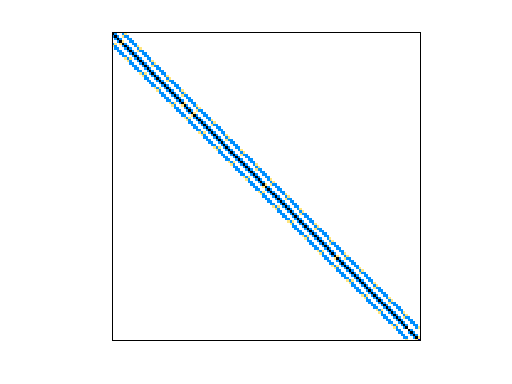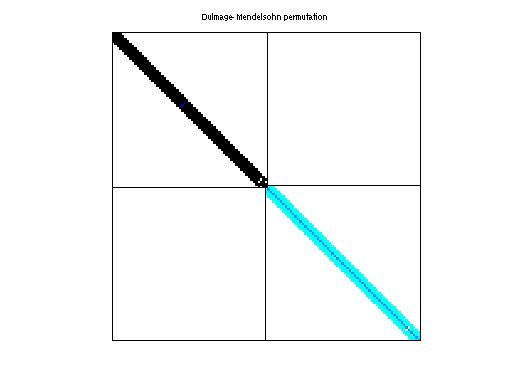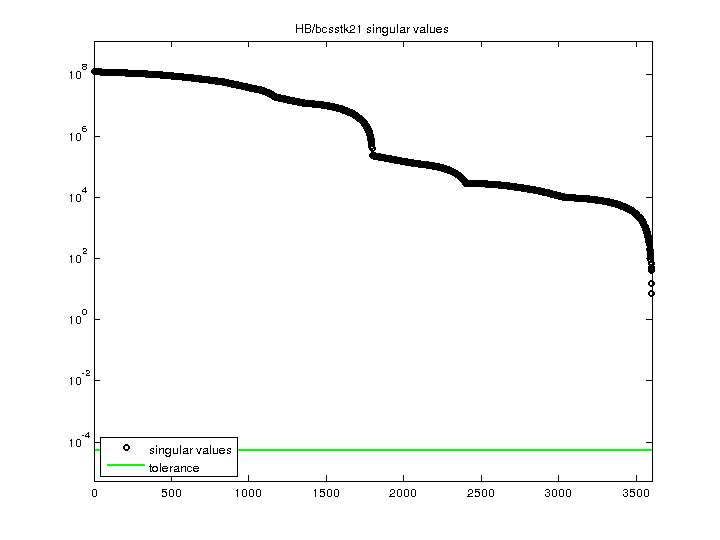Matrix: HB/bcsstk21

Description: SYMMETRIC STIFFNESS MATRIX - CLAMPED SQUARE PLATE(undirected graph drawing)• Matrix group: HB
• download as a MATLAB mat-file, file size: 43 KB. Use UFget(43) or UFget('HB/bcsstk21') in MATLAB.

 Matrix properties number of rows 3,600 number of columns 3,600 nonzeros 26,600 structural full rank? yes structural rank 3,600 # of blocks from dmperm 2 # strongly connected comp. 2 explicit zero entries 0 nonzero pattern symmetry symmetric numeric value symmetry symmetric type real structure symmetric Cholesky candidate? yes positive definite? yes

 author J. Lewis editor I. Duff, R. Grimes, J. Lewis date 1984 kind structural problem 2D/3D problem? yes

 Ordering statistics: result nnz(chol(P*(A+A'+s*I)*P')) with AMD 90,684 Cholesky flop count 4.2e+06 nnz(L+U), no partial pivoting, with AMD 177,768 nnz(V) for QR, upper bound nnz(L) for LU, with COLAMD 159,993 nnz(R) for QR, upper bound nnz(U) for LU, with COLAMD 295,488

 SVD-based statistics: norm(A) 1.27314e+08 min(svd(A)) 7.21415 cond(A) 1.76478e+07 rank(A) 3,600 sprank(A)-rank(A) 0 null space dimension 0 full numerical rank? yes

 singular values (MAT file): click here SVD method used: s = svd (full (A)) ; status: ok